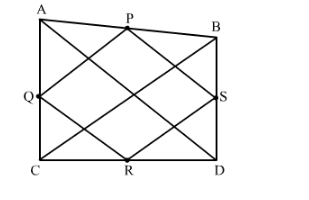# ABCD is a quadrilateral in which AD = BC. If P, Q, R, S`
Question:

ABCD is a quadrilateral in which AD = BC. If P, Q, R, S be the mid-points of AB, AC, CD and BD respectively, show that PQRS is a rhombus.

Solution:

Given:
ABCD is quadrilateral in which AD = BC and P, Q, R, S are the mid points of AB, AC, CD, BD respectively.To Prove:
PQRS is  a rhombus.

Proof:
In ∆ABC, P and Q are the midpoints of the sides AB and AC respectively.
By the Mid point theorem, we get
PQ || BC and PQ = 12BC                                                                  ...(1)
In ∆ADC, Q and R are the midpoints of the sides AC and DC respectively.
By the Mid point theorem, we get